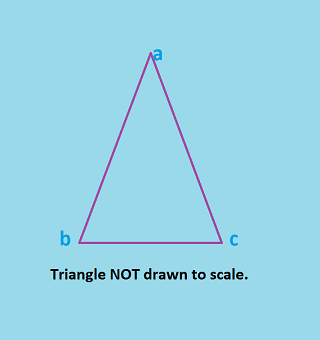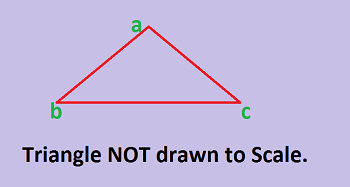# Vertex Angle of an Isosceles Triangle

Instructions:

question 1 of 3

### What does 'a' refer to in this drawing?Create Your Account To Take This Quiz

As a member, you'll also get unlimited access to over 79,000 lessons in math, English, science, history, and more. Plus, get practice tests, quizzes, and personalized coaching to help you succeed.

Try it risk-free for 30 days. Cancel anytime

### 1. If angle 'a' is 10°, what is the measurement of angle 'c?'### 2. In this drawing of a triangle, which side is the base?Create your account to access this entire worksheet
Quizzes, practice exams & worksheets
Certificate of Completion
Create an account to get started

Test your understanding of the vertex angle of isosceles triangles with this worksheet and quiz combo. Topics include what 'a' refers to in a drawing and the measurement of an angle in a given equation.

## Quiz & Worksheet Goals

The worksheet and quiz will get you to:

• Identify the base of a triangle
• Determine how you can remember that an isosceles triangle has two equal sides
• Calculate the measurement of a vertex angle

## Skills Practiced

• Problem solving - use acquired knowledge to solve vertex angle practice problems
• Making connections - use understanding of the concept of the sides of a triangle and how they are connected in an isosceles triangle
• Information recall - access the knowledge you've gained regarding the base of a triangle

Learn more about triangles from the lesson named Vertex Angle of an Isosceles Triangle. Consider the following study points as a guide:

• Real-life triangles
• Example of an isosceles triangle
• Practice problems
Final ExamMath for Kids
Status: Not Started
Chapter ExamShapes for Elementary School
Status: Not Started

Support